### Introduction

Clock jitter is the variation of a clock signal's frequency or period. Either measurement carries the same information, but the period measurement is a simple time interval measurement easily performed using a real-time oscilloscope.

To measure clock jitter as a variation in period, we measure the time interval between each consecutive rising edge at the same crossing threshold over multiple cycles. After measuring the absolute clock signal period, as shown in Figure 1, we plot the track and histogram of the period from cycle to cycle for statistical analysis of the variation.

In the process of making this measurement, we make a series of oscilloscope sample clock tests and timebase adjustments for consistency checks. While measuring jitter is less about absolute accuracy than about the relative precision of measuring the time interval from cycle to cycle, a fundamental part of that is the ensuring the absolute accuracy of the oscilloscope's timebase.

If we have a robust way of measuring clock jitter, we have the basis for measuring the clock signal's sensitivity to other features in the environment that can affect the period. Voltage noise on the power rail is just one external force that can affect clock jitter, which we show you how to measure in the application note, Measuring Clock Jitter Sensitivity to Power Rail Noise.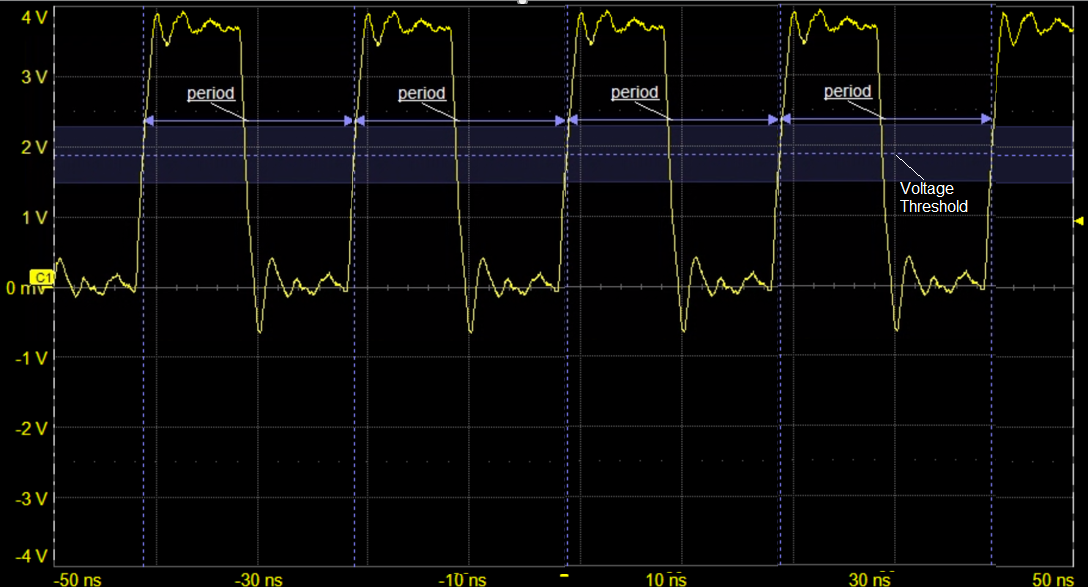Figure 1: Clock jitter measured as the variation of a clock signal's period over multiple cycles.

### Test Equipment

This application note is organized as a step-by-step tutorial that you can perform in your lab using your own clock signal source.

Besides your clock signal source, you will need:

A real-time oscilloscope capable of sampling at least 5x the signal bandwidth based on the signal rise time (bandwidth = 5*(0.35/trise) for oscilloscopes with single-pole frequency response)

We used a WavePro HD 12-bit, 4-Ch, 8 GHz, 20 GS/s, 5 Gpts oscilloscope with 60 fs sample clock jitter to measure a square wave signal between 10 and 66 MHz. We recommend at least a 2-Ch, 2 GHz, 20 Gs/s oscilloscope with track and histogram functions.

Synthesized function generator, or other known frequency signal source, 1 part per million (PPM) frequency accuracy, at least 50 MHz frequency

We used a synthesized function generator with 1 PPM absolute accuracy to generate a 30 MHz, 5 V signal and applied it to the oscilloscope on C1.

Two 50 Ω coaxial cables to input signals from the clock and function generator, or equivalent single-ended probe for your clock line.

The clock source used in our examples is a 5-stage ring oscillator based on the 74AC14 hex inverter powered by a 5 V rail, shown embedded in a PCB in Figure 2. The logical state of the stage 5 output is inverted from the original stage 1 input, resulting in oscillation with a period equal to 10 times the propagation delay of each hex inverter stage. The specified propagation delay for a power level of 5 V is from 1.5 ns to 10 ns, so the clock period is anywhere in the range of 15 ns to 100 ns, for a frequency anywhere from 66 MHz to 10 MHz. This extreme variation is why it is so important to test clock jitter in situ for every application.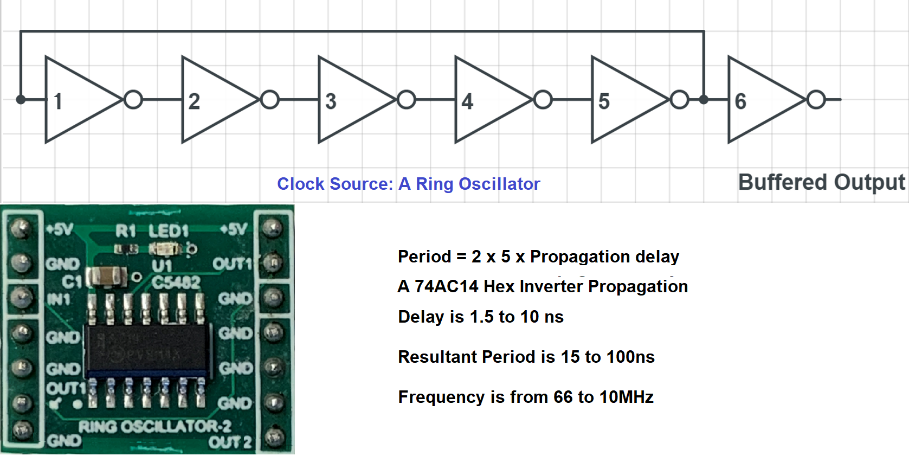Figure 2: 5-stage ring oscillator clock based on the 74AC14 hex inverter.

### Step-by-Step

##### Test the Oscilloscope Intrinsic Sample Clock Jitter

Before measuring the clock signal jitter, it's advisable to do a "situational awareness" test of the oscilloscope's timebase accuracy using a known source to verify it meets the specification.

1. Turn on your known source and clock signal channels.

2. Recall the oscilloscope's default setup to bring it into a known state.

3. Set a 50% Edge trigger on the known source channel.

4. Using parameters with statistics on, measure the signal rise time, frequency and period for about 10,000 cycles.

5. Calculate the fractional uncertainty (Df/f) in PPM as (Df/f)*10^6.

We measure a mean frequency of 30.00028 MHz, Df =280 Hz, so the fractional uncertainty in PPM on a 30 MHz signal is 280/30*10^6 = 9.333, indicating the absolute accuracy of the oscilloscope timebase is under 10 PPM.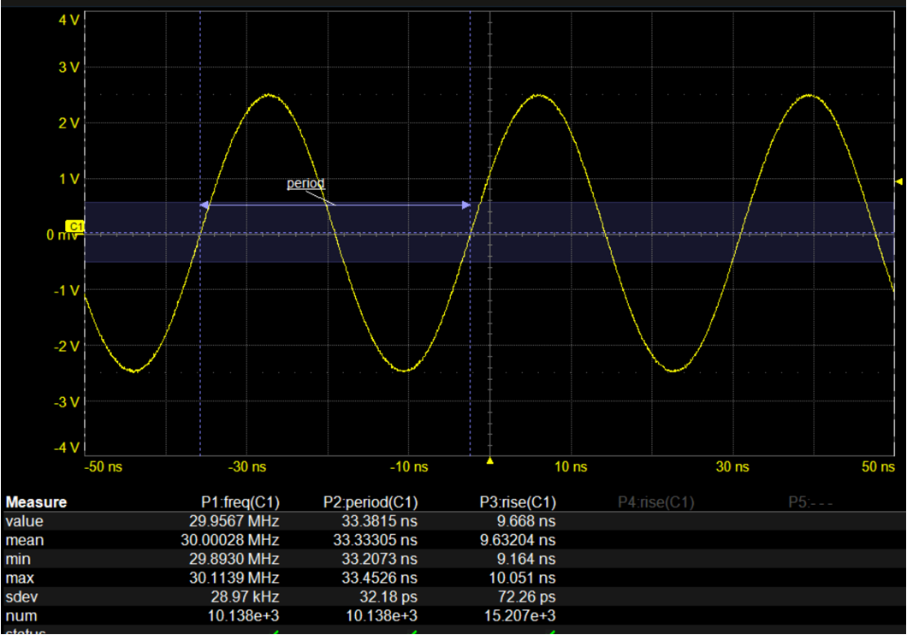Figure 3: Measure the frequency and period of a known source to ascertain oscilloscope's intrinsic sample clock jitter.
##### Estimate the Expected Clock Signal Period Using the Display

6. Set the highest fixed sample rate available on the oscilloscope.

Our sample rate is set to 20 GS/s, the WavePro HD 4-channel maximum.

7. Set a 50% Edge trigger on the clock signal channel.

8. Readjust the V/div of the clock signal channel and the Time/div until two periods of the signal are visible on the graticule.

9. Using the waveform on the display, estimate the clock signal period and rise time.

Figure 4 shows the waveform of the ring oscillator on C2. The period is a little more than four divisions, which at 5 ns/division is about 21 ns. The rise time is a little less than two minor divisions, each of which represents 1 ns, so about 1.5 ns.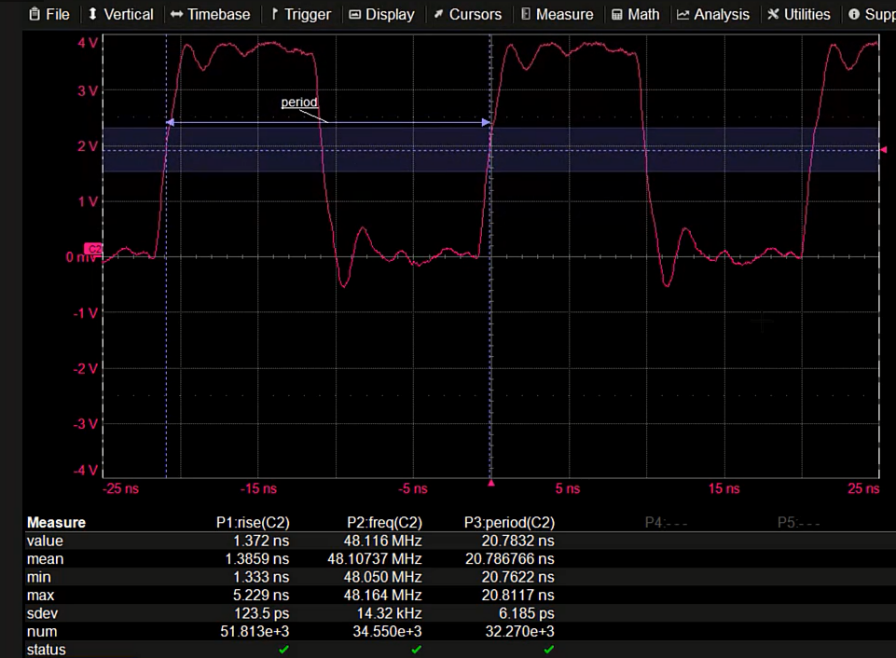Figure 4: Use both the graticule and parameters to measure the clock signal period and rise time.
##### Measure the Clock Signal Period Using Parameters

10. Using parameters with statistics on, measure the rise time, frequency and period of the clock signal.

Tip: On OneTouch oscilloscopes, you can just drag the clock signal descriptor box onto the measurement table cells to switch the measurements from the known source to the clock signal.

With parameters, we measure a mean frequency of 48 MHz, corresponding to a period of 20.75 ns, very close to the estimated 21 ns, and within the specified 10 to 66 MHz. The estimated rise time was 1.5 ns, and the measured value is 1.38 ns.

##### Calculate the Standard Deviation of the Clock Signal Period

The standard deviation (sdev) is a measure of the spread of values about the mean. By definition, in a Gaussian distribution, 68% of all measured values are within ±1 standard deviation from the mean. The period sdev value is a good figure of merit for clock jitter, which is a measure of variation from the mean.

With statistics on, the sdev of every parameter measurement is automatically calculated. Our clock period sdev measurement is 6.18 ps. Given that the oscilloscope sample interval at this timebase is 50 ps, the sdev being lower than the sampling period indicates that interpolation is being applied to the measured values in order to increase the time resolution.

However, since the oscilloscope's intrinsic sample clock jitter is specified at 60 fs, and we have ascertained an accuracy of ~9 PPM, the measured period jitter at 6.18 ps is much higher than the fundamental limit of the oscilloscope.

##### Increase the Timebase to Lengthen the Acquisition

11. Keeping the maximum fixed sample rate, increase the Time/div until you are acquiring a large number of contiguous cycles.

We recommend capturing two to four million samples per acquisition, which is a good balance between processing time and record length.

In our example, we increase to 20 µs/div for a total acquisition time of 200 µs and record length of 4M sample points.

At 4M points per acquisition and over 6.3 million measurements in the buffer, our period measurement still has an sdev of 6.1 ps— around 6 ps every 20 ns period, or about 0.03% variation in the period. (Note: the 5 ns/div zoom of the waveform, which overlays the 20 µs/div waveform in Figure 5, is not required.)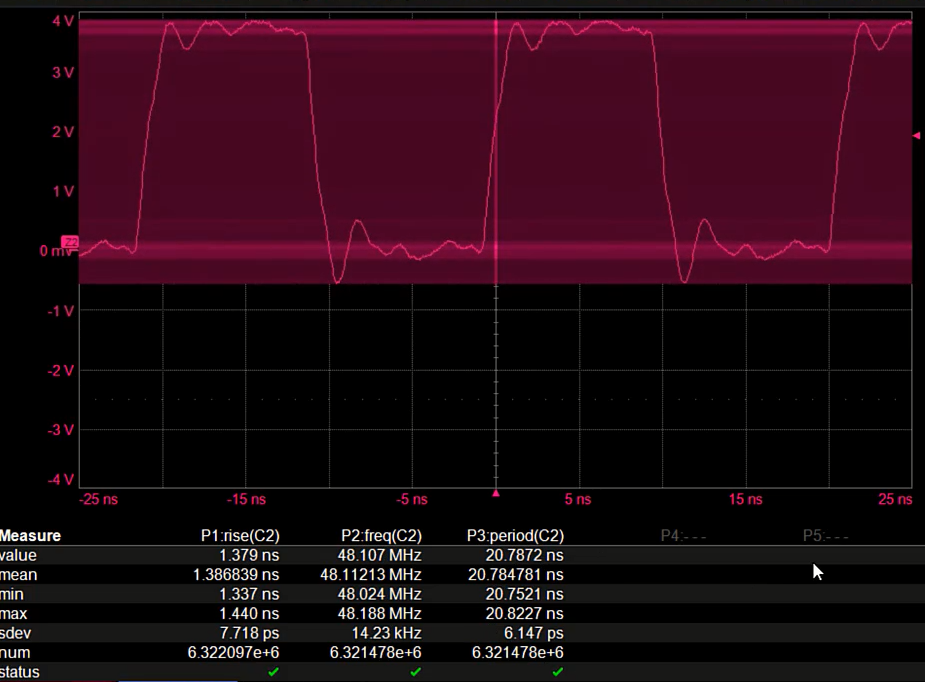Figure 5: The clock signal acquired at 20 µs/div (200 µs record length) overlaid by a zoom of the waveform at 5 ns/div. The parameters are reporting the measurements on the full acquisition.
##### Track and Histogram the Clock Signal Period Measurement

12. Create a track function of the period measurement. You can just click the period cell in the Measure table to open the measurement setup dialog (Pn), then click the Track button on the dialog.

The track plots the measured parameter values synchronous with the acquired waveform. Any variation in the period is mapped to its occurrence on the acquired waveform. Once we have the track, we can perform both statistical and frequency analysis of it to distinguish the “fingerprint” of the normal clock jitter from the “fingerprint” of other jitter sources in the circuit.

13. Create a histogram function of the period measurement. While on the period Pn dialog, click the Histogram button on the dialog.

The Histogram sorts the period values into bins of uniform “width,” or range of values. It then plots a bar chart of the number of values in each bin versus the measured value.

14. Modify the histogram to plot 20,000 Values into 100 Bins of 10 ps Width each. You can click the histogram descriptor box to open the function (Fn) setup dialog, then modify the settings on the Histogram subdialog to the right.

15. Using the graticule, estimate the number of picoseconds in the histogram from edge to edge at center height (full width-half max). Alternatively, create a histogram parameter measuring the full width-half max (not shown here). This should roughly equal the measured period sdev.

Figure 6 shows both the track and the histogram of our period measurement. The track function is displayed over the acquired waveform, while the histogram is plotted in a separate grid.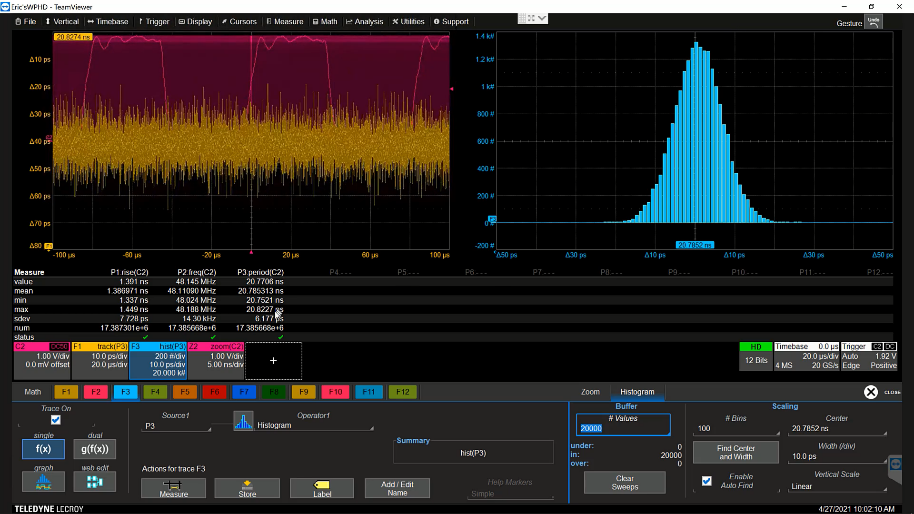Figure 6: Track and histogram of the period measurement. The track shows the variation of period as a function of time, while the histogram is a visual indicator of the measurement distribution.

The vertical scale of our track function is 10 ps/div, same as the horizontal scale of our histogram. Just by “eyeballing” the extent of it, we can confirm the sdev matches the 6 ps sdev that is calculated by the period measurement statistics.

The histogram is centered near the mean value of the period measurement at 20.7852 ns. The saddle shape of the histogram is characteristic of the Gaussian or Normal distribution, a good indication that the clock jitter is the result of a random process. The full width-half max measurement is close to the period sdev of ±6 ps.

##### Consistency Check: Lower the Sample Rate Until the Measurement Degrades

At this point it is worthwhile to investigate how the oscilloscope's sampling rate affects the measured clock jitter. This is done for “situational awareness,” to ensure that the instrumentation is not affecting the measurements.

16. Change the oscilloscope timebase from the maximum sample rate to about half and note any change to the period sdev or the histogram shape.

We reduce from 20 GS/s (50 ps resolution) to 10 GS/s (100 ps resolution) with no significant change.

17. Lower the sample rate by successive steps until the measurement visibly degrades. You can do this by clicking the down arrow button next to the timebase Sampling Rate field.

We step from 5 (200 ps) to 2.5 (400 ps) to 1 GS/s (1 ns). The result is shown in Figure 7.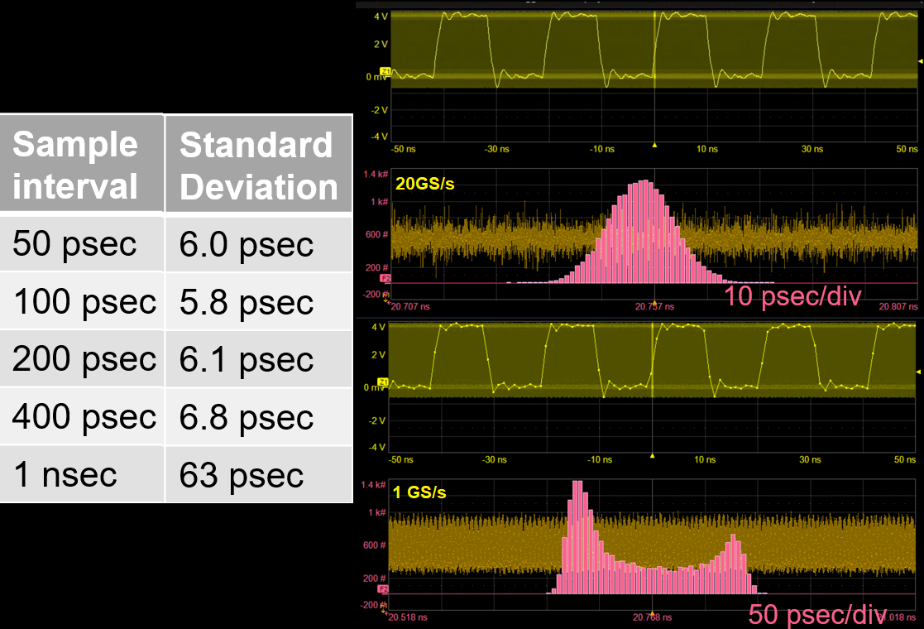Figure 7: Observing the effects of the oscilloscope sampling rate on the clock jitter measurement. Histogram of the period show the significant change in the distribution when the sampling rate drops to 1 GS/s.

The table in Figure 7 shows that the sdev remains close to the originally measured 6 ps until the sample rate drops to 1 GS/s. At 1 GS/s, the edges of the clock signal are not well enough defined by the number of samples to measure the period accurately. There are only about 1.3 samples on the edge (the bright dots on the zoomed clock waveform). As a general rule, you should use the highest possible sampling rate for jitter measurements. However, this confirms that even at a quarter of our maximum sampling rate, measurement accuracy is good, and at 20 GS/s the jitter measurement is quite trustworthy.

### Conclusion

Clock jitter can be measured as the standard deviation (sdev) of the absolute period measurement. Using the oscilloscope display graticule, parameters, tracks and histograms sequentially enables you to cross-examine the data to confirm the measurement. Seeing a consistent result as you push different timebase settings to their limits will confirm whether or not the measurement is trustworthy. For best accuracy when measuring any type of jitter, the oscilloscope sample rate should be as high as possible, and the oscilloscope's specified sample clock jitter must be lower than the measured jitter.

Watch these measurements conducted by Dr. Eric Bogatin in the on-demand webinar, The Impact of Power Rail Noise on Clock Jitter .